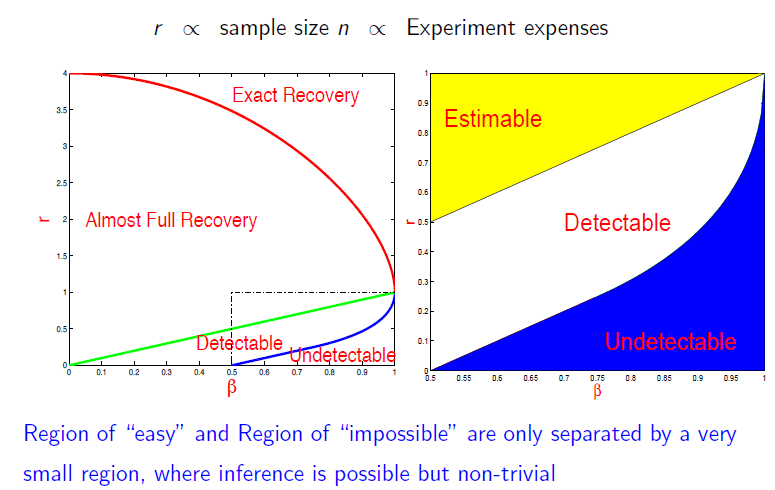# PKU暑期高维统计学习心得[I]

## Higher Criticism and Rare/Weak Signals

Jiashun老师讲关于稀疏、弱信号（rare/weak signals）共三节课，最核心的是Higher Criticism and rare/weak signals，然后还有就是关于变量选择的新思维。

Jiashun老师从FDR的弱点出发引出了自己的思路。

\begin{equation*}
\hat{\mu}_i^H = \left \{
\begin{array}{lc}
y_i, & |y_i| \geq \sigma \cdot t \\
0, & \text{otherwise}
\end{array}
\right.
\end{equation*}

\begin{equation*}
\begin{array}{lr}
H_0 : X_1 \overset{iid}{\sim}N(0, 1), & 1 \leq i \leq p \\
H_1^{(p)}: X_i \overset{iid}{\sim}(1 - \epsilon_p)N(0, 1) + \epsilon N(\tau_p, 1), & 1 \leq i \leq p
\end{array}
\end{equation*}

\begin{equation*}
\begin{array}{lc}
\epsilon_p = p^{-\beta}, & 0.5 < \beta < 1 \\ \tau_p = \sqrt{2r\log p}, & 0 < r < 1 \end{array} \end{equation*}

• $\epsilon_p$很小时，比如$\epsilon_p << 1/\sqrt{p}$时，意味着只有极少的非零均值，此参数刻画着信号稀疏性（$\beta$越大，$\tau_p$越小，信号越越稀疏）；
• $\tau_p$比较小时，信号相对比较弱，此参数刻画着信号的强弱（$r$越大，信号越强）；一般$\tau_p < \sqrt{2 \log p}$时，信号就凑合能用了（only moderate significance）。Higher Criticism，我直译为为高阶鉴别法，Jiashun老师说始于Tukey 1976年Stat 411课程讲义笔记，大师的思维光芒真是能穿越历史呀。Jiashun老师推导的HC与Tukey的略有不同，更为一般化，式子如下：

\begin{equation*}
HC^{*}_p = \max_{0 \leq \alpha \leq \alpha_0}\big\{
\sqrt{p}\big[\frac{\text{fraction significant} at \alpha - \alpha}{\sqrt{\alpha(1 - \alpha)}}\big]
\big\}
\end{equation*}

Jiashun老师说，HC值对强信号、弱信号检测都非常敏感，而FDR仅对强信号敏感。我粗浅地想可能就是HC值基于p值后又做了一次检验的缘故吧。由于没有去做Jiashun老师留下的作业，所以理解还不深刻。后面还是回头再算算Phase Diagram中的边界曲线来加深理解。

Higher Cirticism实施比较简单，过程与FDR过程很类似。步骤如下：

1. 对每个特征都算一个z-score，然后根据z-score算个p值，
2. 对p值排序：$\pi_{(1)} < \pi_{(2)} < \cdots < \pi_{(p)}$ 3. 计算第$k$个HC值，也相当于算了一个z-score：

4. 取最大值，计算相应的$HC^{*}_p$值，找到对应的$k$，前$k$可以认为是真显著的。

Higher Criticism在宇宙学、天文学、基因、异常检测中研究比较多，因为那里的信号比较稀疏和弱，常规的方法已经不能满足需求。另外，HC非常适用于高维的screening、signal detection、classification、clustering等方向，用HC来控制screening中的阈值，比常规的CV、FDR等方法提供了一个新的角度，并且简单有效，无需调参，理论性质也挺好。

P.s. 一不小心突然发现写太多了，本只是记录下心得，不过写着写着觉得还是要重新捋一捋思路才行。之所以写这么多，很大原因是我对penalty太细节化的讨论感到有些厌倦了，里面谈到的统计思想性的东西并不多，所以Jiashun老师东西对我来说比较新颖，便一下子记录了不少，以留作后续继续研读。

Jiashun老师后面还回顾了L0方法的本源，然后说明了在在稀疏、和弱信号下，基于L0而衍生的一系列penalty方法都存在比较大的问题。这个论证让我感觉耳目一新，留作下篇再续。希望后面能在深入了解下Jiashun老师的工作，能够有更深的理解，能跳出当前的状态，既能看到他的方法好处，也能看到他的方法的弱点所在，因为我相信没有一种方法是万能的，总会有不完美的地方。

1. Pingback: PKU暑期高维统计学习心得(II) | 境由心生